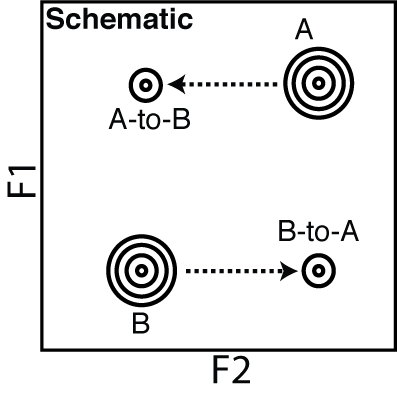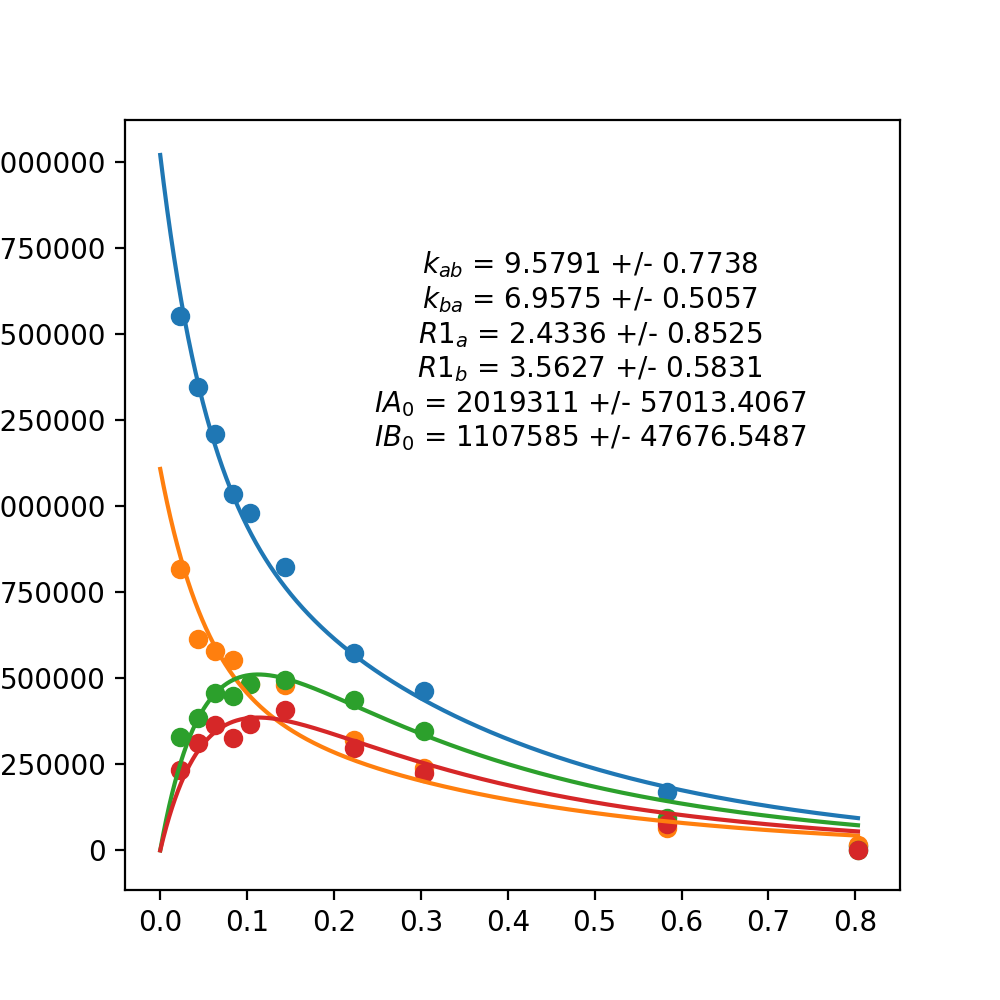Fetching contributors…Cannot retrieve contributors at this time
138 lines (106 sloc) 7.29 KB

# papuaNMR_Exchange

## Nz Exchange Fitting

### Preamble

The fitting program is written in python. I recommend installing the anaconda distribution of python. You will need to use python3 and will also need a few common libraries installed. The libraries you will need are:

• Numpy
• Matplotlib
• SciPy
• Pandas

These python programs are used to perform a fitting of the equations that describe peak intensities as a function of R1 relaxation, exchange rate (k) and exchange (mixing) time between two slowly exchanging conformations. In the Farrow paper below, these confirmations are labeled 'n' and 'u' (native versus unfolded). The nomenclature used for this fitting software is state 'A' and state 'B'. Four peaks appear in these experiments for each exchanging system. The intensity of these four peaks are labeled as:

• IAA: The intensity of system A that is recorded at position A
• IBB: The intensity of system B that is recorded at position B
• IAB: The intensity of system A that has moved to position B
• IBA: The intenisty of system B that has moved to position A

In the experiments described in the references 15N (or F1) is encoded before the exchange period, so it does not change during the mixing period. So the peak for 'A to B' is seen as a peak with the same 15N frequency as A but now has a frequency of B for 1H. This is because the system has now moved to a new 1H position and is recorded directly (F2). See schematic below.### Data Preparation

Data files need to be in CSV format. Example files are included in the 'Example_Data' directory, e.g. H76_noise.csv. This files looks like this:

`````` t,IAA,IAAn,IBB,IBBn,IAB,IABn,IBA,IBAn
0.0232,1553000,49000,816700,49000,329600,49000,233100,49000
0.0432,1345000,49000,613200,49000,385400,49000,312600,49000
0.0632,1208000,47000,580400,47000,458200,47000,364200,47000
0.0832,1036000,45000,552800,45000,447300,45000,325900,45000
0.1032,979300,44000,483200,44000,483600,44000,368200,44000
0.1432,822400,41000,479500,41000,495600,41000,407100,41000
0.2232,572100,37000,319100,37000,437300,37000,297200,37000
0.3032,461800,34000,239900,34000,345300,34000,224200,34000
0.5832,169400,25500,63770,25500,95080,25500,77400,25500
0.8032,13060,23000,16940,23000,10,23000,10,23000
``````

The first line gives titles to the columns - these are required. The remaining lines are exchange time (first column) and intensity data followed by noise estimate for each of the four peaks. Basically, each row is the time and then intensity data for the four peaks of an exchange system at that time point.

Nicely formated for clarity, it looks like this:

t(sec) IAA IAAn IBB IBBn IAB IABn IBA IBAn
0.0232 1553000 49000 816700 49000 329600 49000 233100 49000
0.0432 1345000 49000 613200 49000 385400 49000 312600 49000
0.0632 1208000 47000 580400 47000 458200 47000 364200 47000
0.0832 1036000 45000 552800 45000 447300 45000 325900 45000
0.1032 979300 44000 483200 44000 483600 44000 368200 44000
0.1432 822400 41000 479500 41000 495600 41000 407100 41000
0.2232 572100 37000 319100 37000 437300 37000 297200 37000
0.3032 461800 34000 239900 34000 345300 34000 224200 34000
0.5832 169400 25500 63770 25500 95080 25500 77400 25500
0.8032 13060 23000 16940 23000 10 23000 10 23000

You'll see that each time point has the same noise level. This could be just a single column but I havent implemented that (yet). You also don't have to have the noise estimates, but you will not be able to run Monte Carlo simulations to get an estimate of the accuracy of the fitting parameters.

### Fitting

N.B. - the GUI program does not work right now. Its a work in progress.

The fitting is done with a command line program called PapuaExchange.py. Execute this program with the command:

``````python3 PapuaExchange.py [-h] -data DATA_FILE [-plot PLOT_OUTPUT]
[-mc MONTE_CARLO] [-pdf PDF_PLOT]
``````

Depending on the options, several things will happen. Firstly, in all cases the program will return the fitted parameters if they can be fitted. If the file format is wrong you will mostly get an ugly error. Check the file format. The file with the data is given with the option:

``````-data DATAFILE
``````

where your CSV file name replaces DATAFILE

If you want a plot on the screen of the data and the fit use:

``````-plot 1 or -plot Y
``````

If not, just leave this out or use

``````-plot 0
``````

Note: If you want a pdf file saved of the fitting, you must activate a plot

To name a pdf file for saving data, use:

``````-pdf PDF_PLOT
``````

where PDF_PLOT is the name of the file to save.

If you want to do a Monte Carlo Simulation to estimate how accurate you can model the parameters, use the following command

``````-mc MONTE_CARLO
``````

where MONTE_CARLO is the number of simulations to run. I suggest about 50. If you dont have a noise estimate for your data or you just dont want to do a Monte Carlo simulation just leave this flag out.

### Example commands

You can use the Example Data in the Example_Data directory. For example, a simple fit of the data with a simple return of the parameters would be done like this:

``````python3 PapuaExchange.py -data Example_Data/H76.csv
``````

or

``````python3 PapuaExchange.py -data Example_Data/H76_noise.csv
``````

which will return something like:

``````IAA0: 2018878.1344
IBB0: 1101658.4032
R1A: 2.3819
R1B: 3.5807
k_ab: 9.6044
k_ba: 6.9073
``````

Here, IAA0 and IBB0 are the initial (no mixing/exchange time) estimate of intensity for state A and B, respectively. R1A and R1B is an estimate of the Longtitudinal Relaxation Rate for state A and B, respectively. These numbers have units in the same units of the time numbers given in the data file (usually seconds). k_ab and k_ba are estimates of the rate of exchange from A to B and B to A, respectively. Again, the units of this time parameter is the same as the units used in the data file.

The file H76.csv, which has no noise estimates in it, works fine here because we don't do a Monte Carlo Simulation. But a file with noise esitmates works as well, so long as the first line of the CSV file is accurately labeled.

Now, lets do a full Monte Carlo Simulation with plotting and writing a PDF file. Try:

``````python3 PapuaExchange.py -data Example_Data/H76_noise.csv -mc 50 -plot 1 -pdf H76_Monte_Carlo
``````

There is no need to add a .pdf to the end of a file name, it will be added anyway. This plots on the screen the data and fit and also saves a pdf file called H76_Monte_Carlo.pdf.

It should something like this:References:

Farrow N, Zhang O, Forman-Kay J, Kay L. (1994) A heteronuclear correlation experiment for simultaneous determination of 15N longitudinal decay and chemical exchange rates of systems in slow equilibrium. J. Biomol. NMR. 4(5) 727-34.

Robson, S.A., Peterson, R., Bouchard, L.S., Villareal, V.A., Clubb, R.T. (2010) A heteronuclear zero quantum coherence Nz-exchange experiment that resolves resonance overlap and its application to measure the rates of heme binding to the IsdC protein. J. Am. Chem. Soc. 132, 9522-3.

You can’t perform that action at this time.